# Machine Learning Basics

Before we start this article on machine learning basics, let us take an example to understand the impact of machine learning in the world.

According to a recent article in Forbes,

• Machine learning patents grew at a 34% Compound Annual Growth Rate (CAGR) between 2013 and 2017, the third-fastest growing category of all patents granted.
• International Data Corporation (IDC) forecasts that spending on AI and ML will grow from $12B in 2017 to$57.6B by 2021.

We can safely assume that machine learning has been a dominant force in today’s world and has accelerated our progress in all fields. No matter which industry you look at, machine learning has dramatically altered it. Let’s take an example from the world of trading.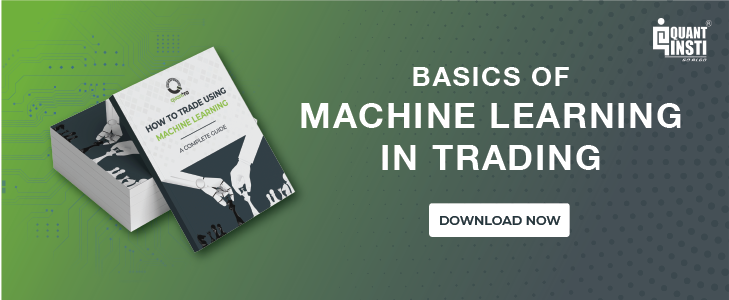Man Group's AHL Dimension programme is a \$5.1 billion dollar hedge fund which is partially managed by AI. After it started off, by the year 2015, its machine learning algorithms were contributing more than half of the profits of the fund even though the assets under its management were far less.

Machine learning has become a hot topic today, with professionals all over the world signing up for ML or AI courses for fear of being left behind.

But exactly what is machine learning? It will be clear to you when you have reached the end of this article. We will cover the following topics in machine learning basics:

## What is Machine Learning?

Machine Learning, as the name suggests, provides machines with the ability to learn autonomously based on experiences, observations and analysing patterns within a given data set without explicitly programming. When we write a program or a code for some specific purpose, we are actually writing a definite set of instructions which the machine will follow.

Whereas in machine learning, we input a data set through which the machine will learn by identifying and analysing the patterns in the data set and learn to take decisions autonomously based on its observations and learnings from the dataset. While Machine learning is a vast topic which will take more than a few articles and courses, today we will focus on machine learning basics, so that you will know what to expect when you dive into machine learning algorithms.

Think of Facebook’s facial recognition algorithm which prompts you to tag photos whenever you upload a photo. Even voice assistants use machine learning to identify and service the user’s request. Tesla’s autopilot feature is another example.

### How do Machines learn?

Well, the simple answer is, just like humans do! First, we receive the knowledge about a certain thing and then keeping this knowledge in mind, we are able to identify the thing in the future. Also, past experiences help us in taking decisions accordingly in the future. Our brain trains itself by identifying the features and patterns in knowledge/data received, thus enabling itself to successfully identify or distinguish between various things.

### Machine Learning timeline

Machine learning is not a recent phenomenon. In fact, neural networks were first introduced as a concept in a research paper in the year 1943!

Although in the early days progress in machine learning was somewhat slow due to the high cost of computing which made this domain only accessible to large academic institutions or multinational corporations. There was also the fact data in itself was difficult to acquire for a company’s needs.

But with the advent of the internet, we are now generating quintillions of data everyday!

Couple that with the reduction in the price of computations and we find that machine learning is a more than a viable proposition.

Let’s try to plot a timeline with some of the notable events in the history of machine learning below: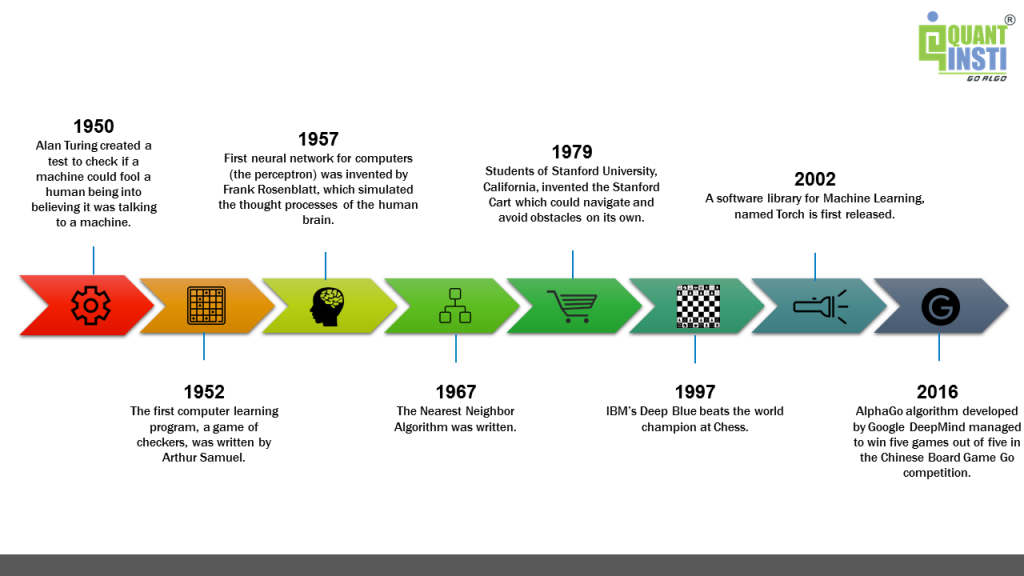## Difference between Machine Learning, Deep Learning, Artificial Intelligence?

While learning about machine learning basics, one often confuses Machine Learning, Artificial Intelligence and Deep Learning. The below diagram clears the concept of machine learning.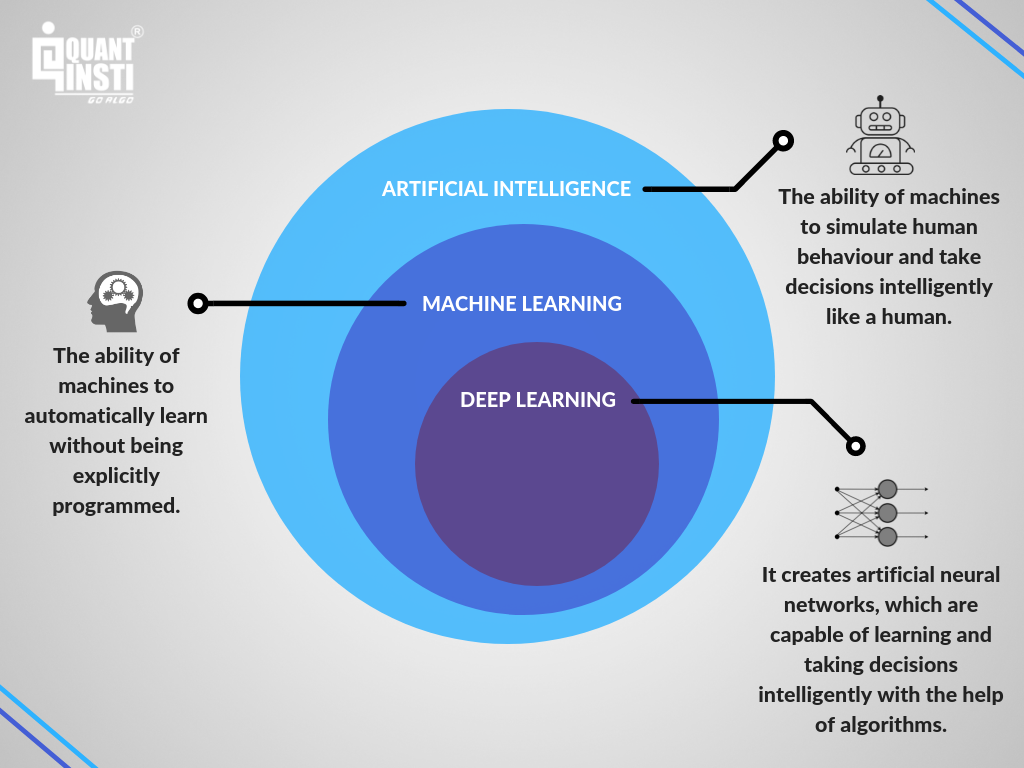We hope that the diagram has helped dispel any doubts you had regarding the three disciplines. Now we move to the heart of the matter.

## Components of Machine Learning

Let’s break down the machine learning process so that we can understand it in detail. We will take a small example as we go through it.

### Collecting and preparing data

The first step in machine learning basics is that we feed knowledge/data to the machine, this data is divided into two parts namely, training data and testing data.

Consider that we want to build software which can identify a person as soon as their photo is shown. We start by collecting data, ie photos of people. Now in this phase, we have to make sure that our data is representative of the entire population ie, if we include only adults from 20 -40 years of age, the software will fail if it is shown a picture of a baby.

The data is usually split into 80/20 or 70/30 to make sure that the model once sufficiently trained can be tested later.

### Choosing and training a model

This is the second step in machine learning basics. We have a variety of machine learning algorithms and models which have been created and modified further so that it can solve a particular type of problem. Thus, it is imperative we choose and train a model depending on its suitability for the problem at hand.

### Evaluating a model

The machine learns the patterns and features from the training data and trains itself to take decisions like identifying, classifying or predicting new data. To check how accurately the machine is able to take these decisions, the predictions are tested on the testing data.

In this case, we will first work on the training data and once the model is sufficiently trained, we use it on the testing data to understand how successful it is in recognising the faces in the photo.

### Hyperparameter tuning and Prediction

In Machine learning terminology, the hyperparameters are parameters that cannot be estimated by the model itself, but we still need to account for them as they play a crucial role in increasing the performance of the model.

Traditionally speaking, hyperparameters in a machine learning model are the parameters which need to be specified by the user, in order to run the algorithm. Classical parameters are learned from the data, hyperparameters may or may not be learned from data.

For example, in a decision tree shown below. The hyperparameters are

1. Number of leaf nodes
2. Depth of tree
3. Minimum sample required to split the node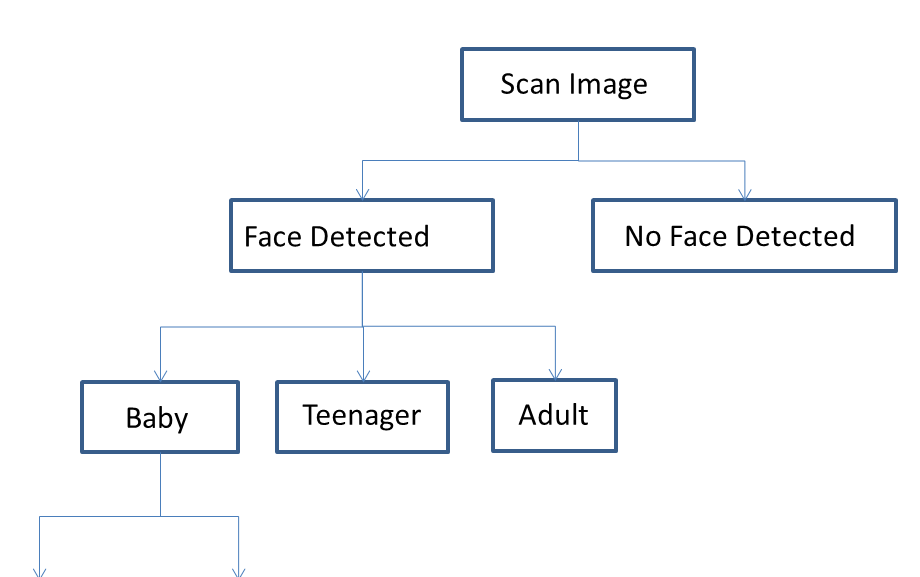A model can have many hyperparameters and the process of finding the best possible combination of hyperparameters is referred to as hyperparameter tuning. Some of the machine learning basics methods for hyperparameter tuning include grid search. Randomised Search, Gradient-based optimisation. To go into detail about these methods would perhaps be overkill as we are concentrating on Machine learning basics, but a general understanding of these processes is enough for now.

Once the hyperparameter optimisation process is completed, we can say that the machine learning model is built and depending on its success rate or rather, the prediction ability, we can deploy it in the real world.

Thus, in this manner, we can build a machine learning algorithm.

Just like every building needs a foundation, we need to import python libraries which will help us build the machine learning algorithm. Let’s go through a few python machine learning libraries now.

## Python Libraries for Machine Learning basics

### Scikit-learn

It is a Python Machine Learning library built upon the SciPy library and consists of various algorithms including classification, clustering and regression, and can be used along with other Python libraries like NumPy and SciPy for scientific and numerical computations. Some of its classes and functions are sklearn.cluster, sklearn.datasets, sklearn.ensemble, sklearn.mixture etc.

### TensorFlow

TensorFlow is an open-source software library for high-performance numerical computations and machine learning applications such as neural networks. It allows easy deployment of computation across various platforms like CPUs, GPUs, TPUs etc. due to its flexible architecture. Learn how to install TensorFlow GPU here.

### Keras

Keras is deep learning library used to develop neural networks and other deep learning models. It can be built on top of TensorFlow, Microsoft Cognitive Toolkit or Theano and focuses on being modular and extensible.

We have now laid the groundwork and covered most of the machine learning basics till now. Let’s move further and understand a few machine learning algorithms.

## Types of Machine Learning Algorithms

Machine Learning algorithms can be classified into:

1. Supervised Algorithms - Linear Regression, Logistic Regression, KNN classification, Support Vector Machine (SVM), Decision Trees, Random Forest, Naive Bayes’ theorem
2. Unsupervised Algorithms - K Means Clustering.
• Reinforcement Algorithm

Let us dig a bit deeper in these machine learning basics algorithms

### Supervised Machine Learning Algorithms

In this type of algorithm, the data set on which the machine is trained consists of labelled data or simply said, consists both the input parameters as well as the required output.

Let’s take the previous example of facial recognition and once we have identified the people in the photos, we will try to classify them as baby, teenager or adult. Here baby, teenager and adult will be our labels and our training dataset will already be classified into the given labels based on certain parameters through which the machine will learn these features and patterns and classify some new input data based on the learning from this training data.

Supervised Machine Learning Algorithms can be broadly divided into two types of algorithms; Classification and Regression.

#### Classification Algorithms

Just as the name suggests, these algorithms are used to classify data into predefined classes or labels. We will discuss one of the most used classification algorithm known as the K-Nearest Neighbor (KNN) Classification Algorithm.

KNN Classification Machine Learning Algorithm

This algorithm is used to classify a set of data points into specific groups or classes based on the similarities between the data points. Let’s consider an example where we need to check whether a person is fit or not based on the height and weight of a person. Suppose we give the following table as the training data set: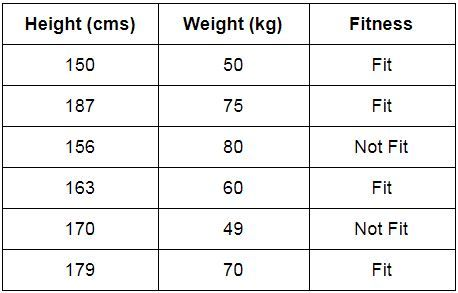Now consider a new person needs to be classified as fit/not fit. Let us consider the value of K=3, which means will consider 3 nearest neighbours. The nearest neighbours can be found out by determining the Euclidean difference between the height and weight of one person and the height and weight of the persons given in the table. The persons with the 3 least differences will be considered as the nearest neighbours. Now we will check how many out of these 3 are fit. If 2 or more out of the 3 are fit, then we will classify the new person as fit and vice versa. In case, we get an equal number of neighbours with different outcomes, then we can increase the value of K and check again.

KNN learns as it goes, in this sense, it does not need an explicit training phase and starts classifying the data points decided by a majority vote of its neighbours.

The object is assigned to the class which is most common among its k nearest neighbours.

Another way to explain the KNN Machine learning classification algorithm is in the following manner:

Let’s consider the task of classifying a green circle into class 1 and class 2. Consider the case of KNN based on the 1-nearest neighbour. In this case, KNN will classify the green circle into class 1. Now let’s increase the number of nearest neighbours to 3 i.e., 3-nearest neighbour. As you can see in the figure there are ‘two’ class 2 objects and ‘one’ class 1 object inside the circle. KNN will classify a green circle into class 2 object as it forms the majority.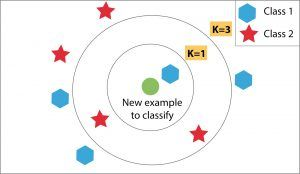#### Regression Machine Learning Algorithms

These algorithms are used to determine the mathematical relationship between two or more variables and the level of dependency between variables. These can be used for predicting an output based on the interdependency of two or more variables.

For example, an increase in the price of a product will decrease its consumption, which means, in this case, the amount of consumption will depend on the price of the product. Here, the amount of consumption will be called as the dependent variable and price of the product will be called the independent variable. The level of dependency on the amount of consumption on the price of a product will help us predict the future value of the amount of consumption based on the change in prices of the product.

We have two types of regression algorithms: Linear Regression and Logistic Regression

Linear Regression Machine Learning

Initially developed in statistics to study the relationship between input and output numerical variables, it was adopted by the machine learning community to make predictions based on the linear regression equation.

The mathematical representation of linear regression is a linear equation that combines a specific set of input data (x) to predict the output value (y) for that set of input values. The linear equation assigns a factor to each set of input values, which are called the coefficients represented by the Greek letter Beta (β).

The equation mentioned below represents a linear regression model with two sets of input values, x1 and x2. y represents the output of the model, β0, β1 and β2 are the coefficients of the linear equation.

y = β0 + β1x1 + β2x2

When there is only one input variable, the linear equation represents a straight line. For simplicity, consider β2 to be equal to zero, which would imply that the variable x2 will not influence the output of the linear regression model. In this case, the linear regression will represent a straight line and its equation is shown below.

y = β0 + β1x1

A graph of the linear regression equation model is as shown below: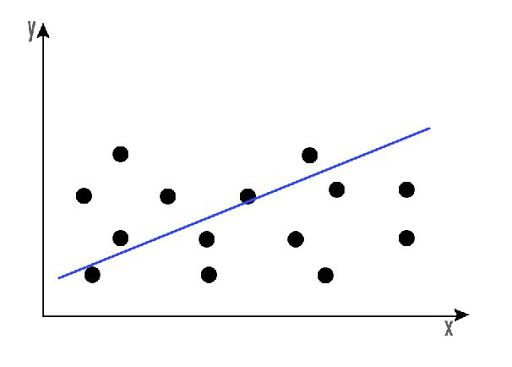Linear regression can be used to find the general price trend of a stock over a period of time. This helps us understand if the price movement is positive or negative.

You can learn about Linear Regression and how it can be used to predict the stock prices in detail in this blog.

Logistic Regression Machine Learning Algorithm

In logistic regression, our aim is to produce a discrete value, either 1 or 0. This helps us in finding a definite answer to our scenario.

Logistic regression can be mathematically represented as,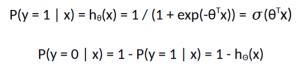The logistic regression model computes a weighted sum of the input variables similar to the linear regression, but it runs the result through a special non-linear function, the logistic function or sigmoid function to produce the output y.

The sigmoid/logistic function is given by the following equation.

y = 1 / (1+ e-x)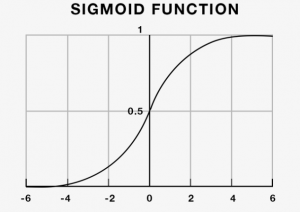In simple terms, logistic regression can be used to predict the direction of the market.

So far, the machine learning algorithms explained above were exclusively classification or regression-based algorithms. Now we will look at certain Supervised machine learning algorithms which can be both.

#### Support Vector Machine (SVM) Learning Algorithm

Support Vector Machine was initially used for data analysis. Initially, a set of training examples is fed into the SVM algorithm, belonging to one or the other category. The algorithm then builds a model that starts assigning new data to one of the categories that it has learned in the training phase.

In the SVM algorithm, a hyperplane is created which serves as a demarcation between the categories. When the SVM algorithm processes a new data point and depending on the side on which it appears it will be classified into one of the classes.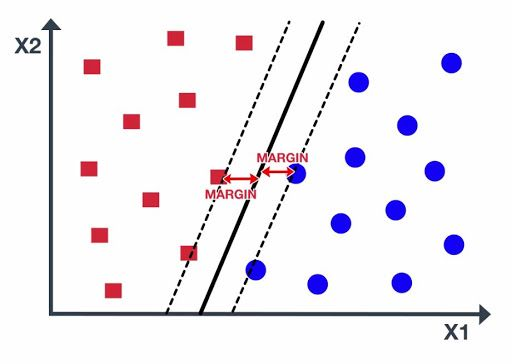When related to trading, an SVM algorithm can be built which categorises the equity data as a favourable buy, sell or neutral classes and then classifies the test data according to the rules.

#### Decision Trees

Decision trees are basically a tree-like support tool which can be used to represent a cause and its effect. Since one cause can have multiple effects, we list them down (quite like a tree with its branches).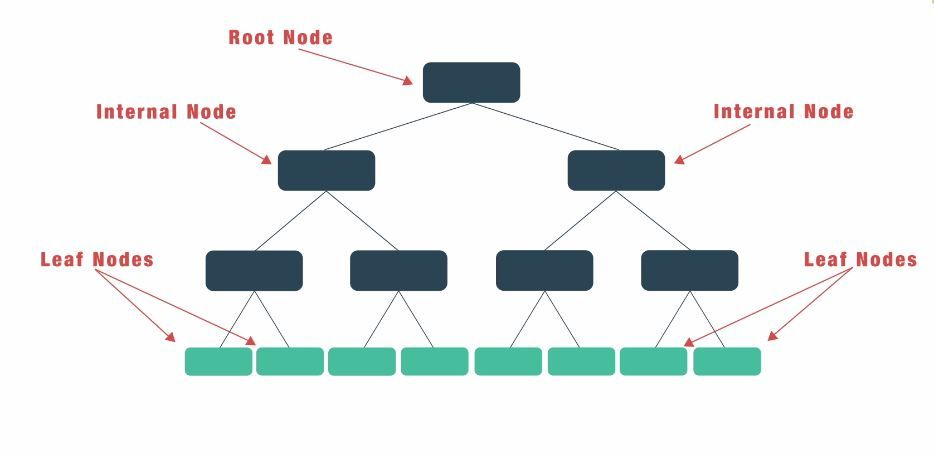We can build the decision tree by organising the input data and predictor variables, and according to some criteria that we will specify.

The main steps to build a decision tree are:

1. Retrieve market data for a financial instrument.
2. Introduce the Predictor variables (i.e. Technical indicators, Sentiment indicators, Breadth indicators, etc.)
3. Setup the Target variable or the desired output.
4. Split data between training and test data.
5. Generate the decision tree training the model.
6. Testing and analyzing the model.

The disadvantage of decision trees is that they are prone to overfitting due to their inherent design structure.

#### Random Forest

A random forest algorithm was designed to address some of the limitations of decision trees.

Random Forest comprises of decision trees which are graphs of decisions representing their course of action or statistical probability. These multiple trees are mapped to a single tree which is called Classification and Regression (CART) Model.

To classify an object based on its attributes, each tree gives a classification which is said to “vote” for that class. The forest then chooses the classification with the greatest number of votes. For regression, it considers the average of the outputs of different trees.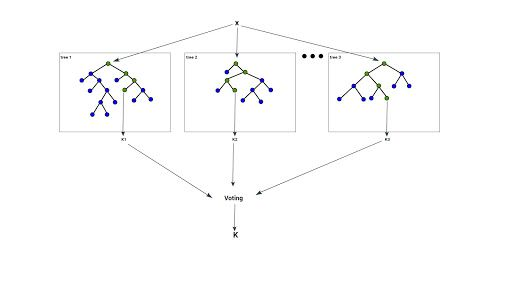Random Forest works in the following way:

1. Assume the number of cases as N. A sample of these N cases is taken as the training set.
2. Consider M to be the number of input variables, a number m is selected such that m < M. The best split between m and M is used to split the node. The value of m is held constant as the trees are grown.
3. Each tree is grown as large as possible.
4. By aggregating the predictions of n trees (i.e., majority votes for classification, the average for regression), predict the new data.

#### Naive Bayes theorem

Now, if you remember basic probability, you would know that Bayes theorem was formulated in a way where we assume we have prior knowledge of any event that related to the former event.

For example, to check the probability that you will be late to the office, one would like to know if you face any traffic on the way.

However, the Naive Bayes classifier algorithm assumes that two events are independent of each other and thus, this simplifies the calculations to a large extent. Initially thought of nothing more than an academic exercise, Naive Bayes has shown that it works remarkably well in the real world as well.

Naive Bayes algorithm can be used to find simple relationships between different parameters without having complete data.

We will now look at the next type of Machine learning algorithms, ie Unsupervised machine learning algorithms.

### Unsupervised Machine Learning Algorithms

Unlike supervised learning algorithms, where we deal with labelled data for training, the training data will be unlabelled for Unsupervised Machine Learning Algorithms. The clustering of data into a specific group will be done on the basis of the similarities between the variables. Some of the unsupervised machine learning algorithms are K-means clustering, neural networks. Let us look at the K-means clustering machine learning algorithm.

#### K-means clustering Machine Learning Algorithm

Before we understand the working of the K-means clustering algorithm, let us first break down the word K-means clustering to understand what it means.

Clustering: In this algorithm, we form clusters which are a collection of data points grouped together due to their similarities.

K refers to the number of centroids which will be considered for a specific problem whereas ‘means’ refers to a centroid which is considered as the central point of any cluster.

Working of K-means Clustering Algorithm

1. Define the value of K. For eg: if K= 2, then we will have two centroids.
2. Randomly select K data points as centroids.
3. Check the distance of each data point with the centroids.
4. Assign the data point to the centroid with which it has a minimum distance, thus forming a cluster of similar data points.
5. Recalculate the centroid of each newly formed cluster and reassign the data points to the cluster whose centroid is at a minimum distance from the data point.

You can decide the number of iterations for repeating step 5 to optimize the algorithm. When the centroid stops changing or remains the same after some amount of iterations then that will be our stopping point and the algorithm will be fully optimized.

A simple example would be that given the data of football players, we will use K-means clustering and label them according to their similarity. Thus, these clusters could be based on the strikers preference to score on free kicks or successful tackles, even when the algorithm is not given pre-defined labels to start with.

K-means clustering would be beneficial to traders who feel that there might be similarities between different assets which cannot be seen on the surface.

While we did mention neural networks in unsupervised machine learning algorithms, it can be debated that they can be used for both supervised as well as unsupervised learning algorithms. Let’s understand Artificial and Recurrent Neural networks now.

#### Artificial Neural Network

In our quest to play God, an artificial neural network is one of our crowning achievements. We have created multiple nodes which are interconnected to each other, as shown in the image, which mimics the neurons in our brain. In simple terms, each neuron takes in information through another neuron, performs work on it, and transfers it to another neuron as output.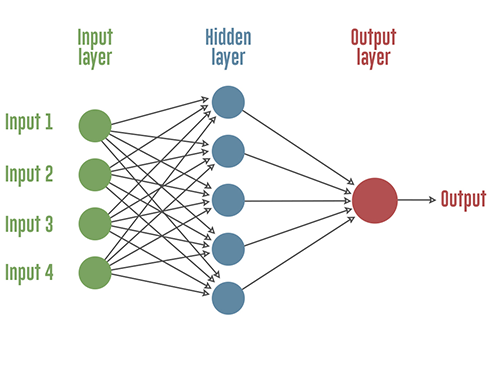Each circular node represents an artificial neuron and an arrow represents a connection from the output of one neuron to the input of another.

Neural networks can be more useful if we use it to find interdependencies between various asset classes, rather than trying to predict a buy or sell choice.

#### Recurrent Neural Networks (RNN)

Did you know Siri and Google Assistant use RNN in their programming? RNNs are essentially a type of neural network which has a memory attached to each node which makes it easy to process sequential data i.e. one data unit is dependent on the previous one.

A way to explain the advantage of RNN over a normal neural network is that we are supposed to process a word character by character. If the word is “trading”, a normal neural network node would forget the character “t” by the time it moves to “d” whereas a recurrent neural network will remember the character as it has its own memory.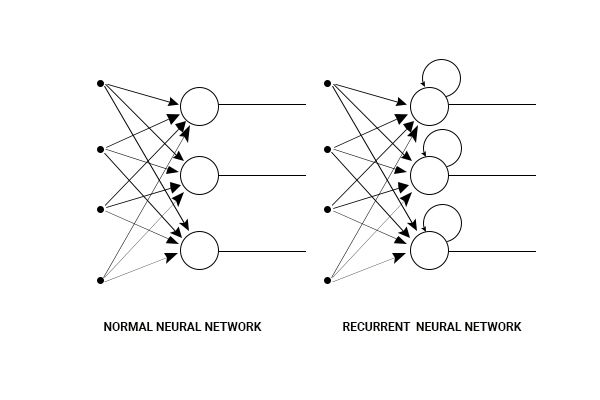### Reinforcement Machine Learning Algorithms

Reinforcement Learning is a type of Machine Learning in which the machine is required to determine the ideal behaviour within a specific context, in order to maximize its rewards. It works on the rewards and punishment principle which means that for any decision which a machine takes, it will be either be rewarded or punished. Thus, it will understand whether or not the decision was correct. This is how the machine will learn to take the correct decisions to maximize the reward in the long run.

For reinforcement algorithm, a machine can be adjusted and programmed to focus more on either the long-term rewards or the short-term rewards. When the machine is in a particular state and has to be the action for the next state in order to achieve the reward, this process is called the Markov Decision Process.

A more technical explanation of the Reinforcement Learning problem can be explored as follows:

The environment is modelled as a stochastic finite state machine with inputs (actions sent from the agent) and outputs (observations and rewards sent to the agent):

• State transition function P(X(t)|X(t-1),A(t))
• Observation (output) function P(Y(t) | X(t), A(t))
• Reward function E(R(t) | X(t), A(t))
• State transition function: S(t) = f (S(t-1), Y(t), R(t), A(t))
• Policy/output function: A(t) = pi(S(t)))

The agent's goal is to find a policy and state-update function so as to maximize the expected sum of discounted rewards

E [ R_0 + g R_1 + g^2 R_2 + ...] = E sum_{t=0}^infty gamma^t R_t

Where, 0 <= gamma <= 1 is a discount factor, which models the fact that future reward is worth less than the immediate reward.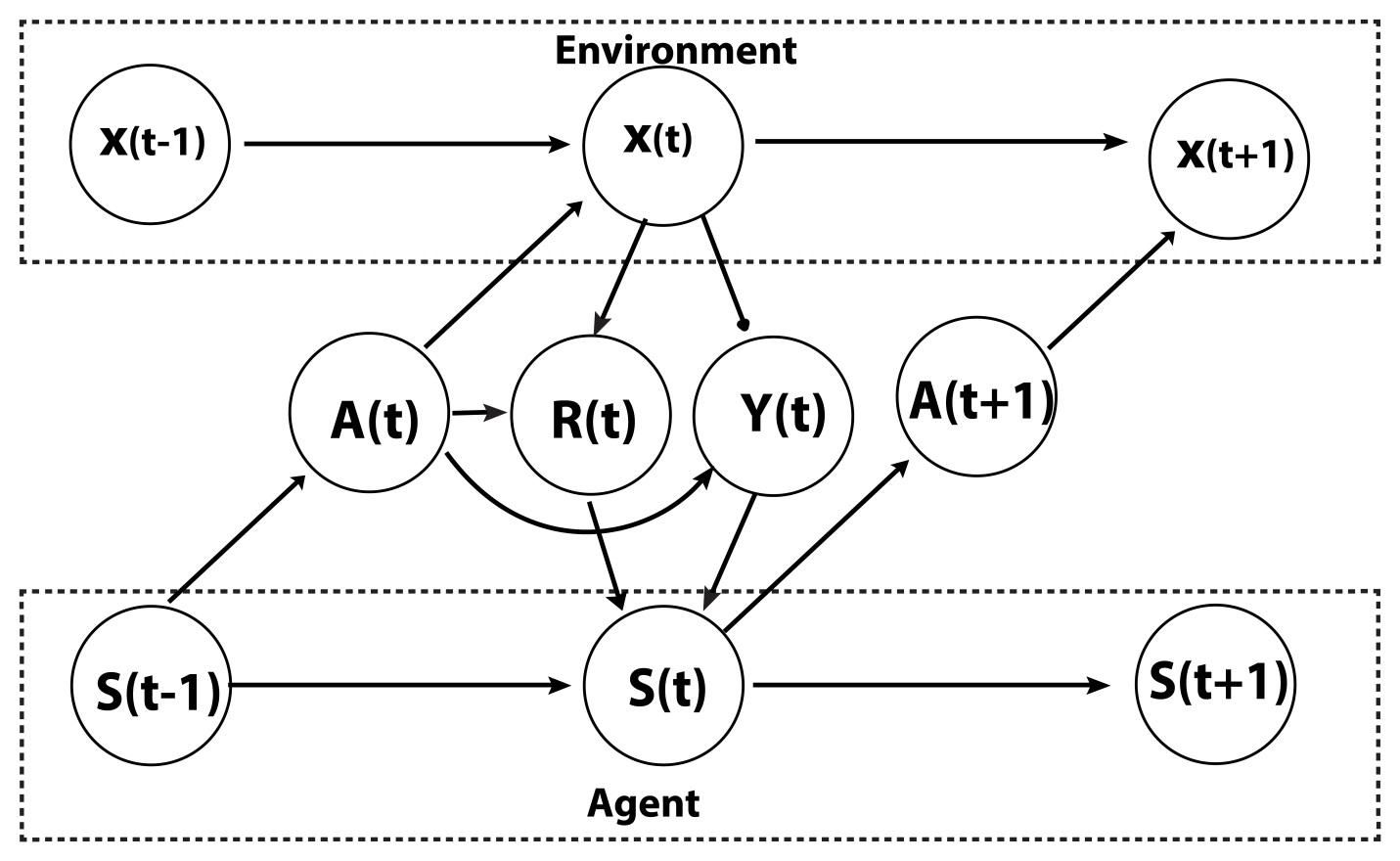The Reinforcement Learning problem requires clever exploration mechanisms. Selection of actions with careful reference to the probability of an event happening is required so that the desired results can be obtained. Further, other drawbacks also make Reinforcement Learning a challenge for the practitioners. Firstly, it turns out to be memory expensive to store the values of each state, as the problems can be very complex. Moreover, problems are also generally very modular; similar behaviours reappear often. Also, limited perception can contribute to the limitations of Reinforcement Learning.

We have now covered most of the popular machine learning algorithms which are used today. As you have understood them, it is imperative that we go through a few terms to make sure we are well versed with machine learning basics.

## Common terms in machine learning basics

Here are a few machine learning basics terms which would be of help as you start your journey in machine learning algorithms.

### Bias

A machine learning model is said to have low bias if its predictability level is high. In other words it makes fewer mistakes when it is working on a dataset.

Bias plays an important role when we have to compare two machine learning algorithms for the same problem statement.

### Cross-validation bias

Cross-validation in machine learning is a technique that provides an accurate measure of the performance of a machine learning model. This performance will be closer to what you can expect when the model is used in a future unseen dataset.

The application of the machine learning models is to learn from the existing data and use that knowledge to predict future unseen events. The cross-validation in machine learning model needs to be thoroughly done to profitably trade in live trading.

You can learn how to perform cross-validation on a machine learning model by going through this article.

### Underfitting

If a machine learning model is not able to predict with a decent level of accuracy, then we say that the model underfits. This could be due to a variety of reasons, including, not selecting the correct features for the prediction, or simply the problem statement is too complex for the selected machine learning algorithm.

### Overfitting

In both machine learning and statistics, overfitting occurs when the model fits the data too well or simply put when the model is too complex. Overfitting model learns the detail and noise in the training data to such an extent that it negatively impacts the performance of the model on new data/test data.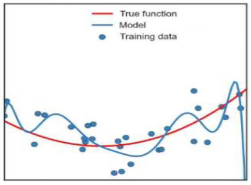Overfitting problem can be solved by decreasing the number of features/inputs or by increasing the number of training examples to make the machine learning algorithms more generalized. The more common way of solving the overfitting problem is by regularization.

These were a few terms we discussed in Machine learning basics. Most of the popular machine learning algorithms are mentioned above. While we could have ended the article here, we thought of going into more detail on one of the hot topics of today ie deep learning. Usually, a neural network consists of three layers, input, hidden layer, and output layer. While the conventional neural network is good enough for solving a lot of problem statements, researchers realised that adding more hidden layers can help us build complex models in an effort to solve different types of complex problems. This is deep learning in a nutshell.

## Difference between Machine Learning and Deep Learning

Machine Learning models lack the mechanism to identify errors, in such cases the programmer needs to step in to tune the model for more accurate decisions, whereas deep learning models can identify the inaccurate decision and correct the model on its own without human intervention.

But for doing so, deep learning models require a huge amount of data and information, unlike Machine Learning models.

## Working of Deep Neural Network

The deep neural network gets its name due to a high number of layers in the networks. Let us now understand what these layers are and how are they used in the deep neural network to give a final output by referring to the diagram given below: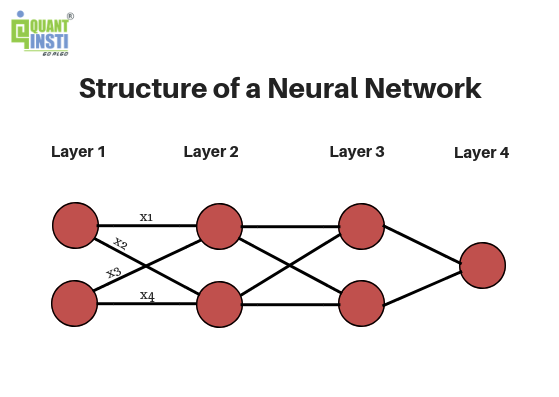### Layers in Deep Neural Network

By looking at this diagram, we see that there are 4 layers present in this deep neural network namely Layer 1, Layer 2, Layer 3 and Layer 4. Every deep neural network consists of three types of layers, which are:

Input Layer (Layer 1): This layer is the first layer in a deep neural network and it provides the input parameters required to process the information. It simply passes these parameters to the further layers without any computation at this layer.

Hidden Layers (Layers 2 and 3): These layers in the deep neural network perform the necessary computations on the inputs received from the previous layers and pass on the result to the next layer. It is crucial to decide the number of layers and the number of neurons in each layer so as to increase the efficiency of the deep neural network. More the number of hidden layers, deeper is the network.

Output Layer (Layer 4): This layer in the deep neural network gives us the final output after receiving the results from the previous layers.

Now that we have understood the types of layers present in a network, let's learn how these layers actually function and give the output data.

Each neuron is connected to all the neurons in the next layer and all these connections have some weights associated with them. But what are these weights and why are they used?

### Weights in Deep Neural Network

Weights, as the name suggests, are used to attach some weightage to a certain feature. Some features might be more important than other features to get the desired output.

For example, close prices and SMAs of the previous days will be considered as more important features than high or low prices while predicting the stock prices for the next day, this will affect the weights attached to these parameters.

These weights are used to calculate the weighted sum for each neuron. x1, x2, x3, x4 represent the weights associated with the corresponding connections in the deep neural network.

Along with the weights, each hidden layer has an activation function associated with it.

### Activation Function in Deep Neural Network

Activation functions decide whether a neuron should be activated or not based on their weighted sum. These are also used to introduce non-linearity by using functions like sigmoid and tanh thus allowing computations for more complex tasks. Without the activation function, the deep neural network would act as a simple linear regression model.

Here are examples of a few activation functions which are used:

• Tanh: Avoids bias in gradients
• Rectified Linear Unit (ReLU): Used for Image Processing
• Softmax: To retain the relevance of outliers

In addition to this, we also add a ‘bias’ neuron to each layer to enable moving the activation function along the x-axis to the left or to the right thus allowing us to fit the activation function better. The bias term which is a constant term also acts as an output whenever the input is absolute zero.

### Processing of Deep Neural Network

The processing starts by calculating the weighted sums for each neuron in the first hidden layer using the inputs received from the input layer. The weighted sums are the sum of the products of the input with the corresponding weights for each connection.

The activation function corresponding to each layer then acts upon these weighted sums to give a final output. This process can also be known as forward propagation.

Once the processing is completed, the predicted output is compared with the actual output to determine the error or loss. For a deep neural network to work accurately, this loss function must be minimized so that the predicted output is as close to the actual output as possible. As we initially choose random weights for the connections in the deep neural network, they might not be the best choice.

Hence, to minimize the loss function, we need to adjust the weights and biases to get accurate results. Backpropagation is the process used to tune the weights and biases such that we get the optimal values of weights and biases thus giving us higher accuracy in our results.

### Deep Learning Applications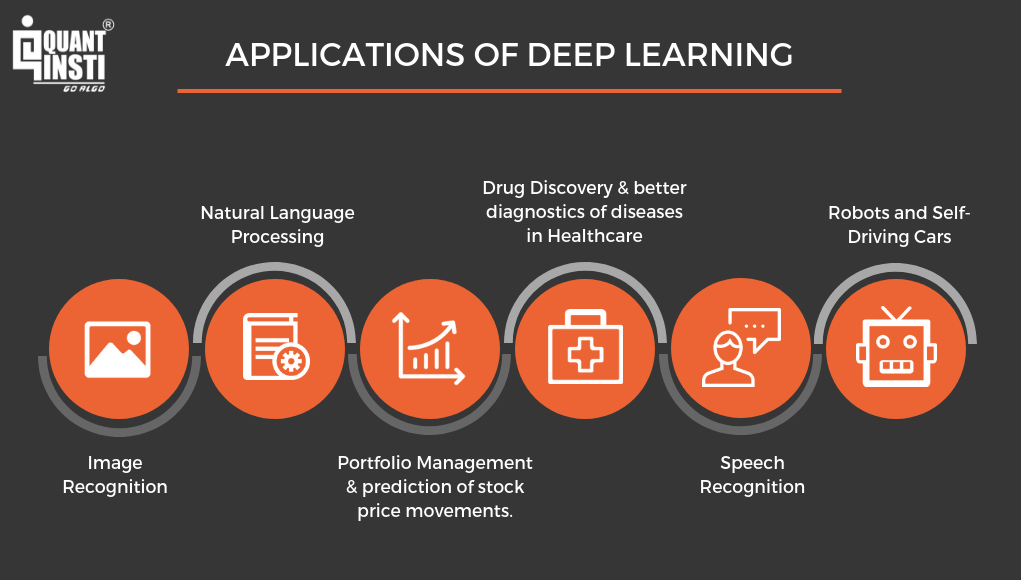We have to remember that Deep learning is actually a subset of Machine learning and thus there will be an overlap between the two when it comes to their applications.

## Applications of Machine Learning

We have covered most about machine learning basics that would clear fundamentals of machine learning, the machine learning process, machine learning concepts and examples of machine learning that would be essential to a machine learning beginner.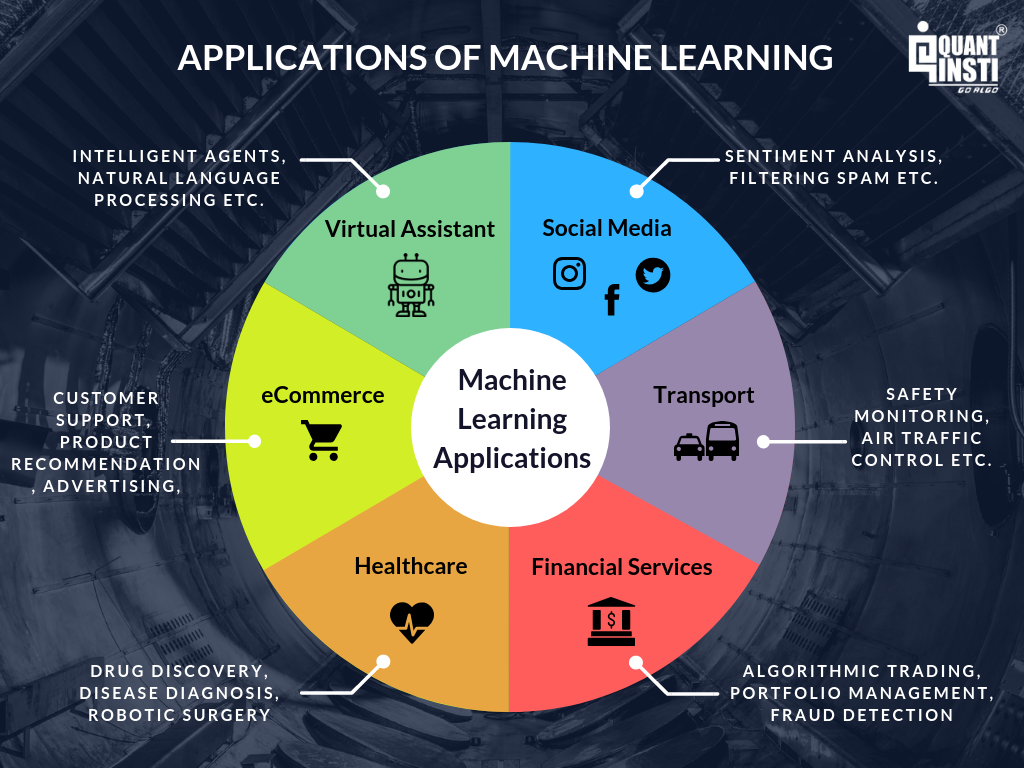As we can observe from the above image, machine learning has a myriad number of applications and is being used in almost all the major fields. Similarly, machine learning has gained huge traction in the field of trading as well with domains such as Algorithmic Trading are witnessing exponential growth. Machine learning for trading is eventually automating the process of trading, wherein the machines themselves are becoming capable to learn from the previous data and take decisions to maximize profit or minimize loss.

Trading strategies, too, can be implemented through machine learning algorithms to optimize the trading process. Some of the open-source machine learning technologies used include TensorFlow, Keras, Scikit-learn, Microsoft Cognitive Toolkit etc.

If you are looking to learn how machine learning can be used for trading, then here is a comprehensive course on Machine Learning for Trading that covers machine learning basics for trading, and it not only consists of video lectures but also provides an interactive platform to practice coding and starts right from the machine learning basics to advanced concepts of machine learning.

## Growth and Future of Machine Learning

Machine Learning is growing at a tremendous rate and we will soon be able to see its applications across all of the major domains. Various reports regarding machine learning have all pointed to an upward growth curve for this domain. According to IFI Claims Patent Services (Patent Analytics), Machine Learning patents witnessed a growth of 116% CAGR between 2017 and 2018, of which the major patent producers included companies like IBM, Microsoft, Intel, Samsung, Google etc.

A survey by MIT and Google Cloud demonstrates that 60% of the organisations have already been using Machine Learning strategies and one-third of them are at an early stage of development. This report by Forrester predicts huge growth for Machine Learning, which forecasts that the Predictive Analytics and Machine Learning (PAML) market will grow at 21% CAGR through 2021.

According to a study by Preqin, 1,360 quantitative funds are known to use computer models in their trading process, representing 9% of all funds. Firms organise cash prizes for an individual's machine learning strategy if it makes money in the test phase and in fact, invest their own money and take it in the live trading phase. Thus, in the race to be one step ahead of the competition, everyone, be it billion dollar hedge funds or the individual trade, all are trying to understand and implement machine learning in their trading strategies. Companies are encouraging their employees to start learning machine learning basics.

Businesses and other major domains are not just adopting new technologies but are adopting new machine learning technologies to automate many of the processes which are helping them increase their productivity. We are now entering into the age of Artificial Intelligence and Machine Learning, thus, making it a domain impossible to ignore and a lot to explore!

## Conclusion

In this article, we have understood machine learning basics as well as the different types of machine learning algorithms used by professional traders in Python. We also know that machine learning is becoming indispensable to the trading world and will become an integral part of the trader’s work life in the years to come.